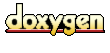Main Page   Class Hierarchy   Compound List   File List   Compound Members   File Members

# Wavelet Packet Transform and Lossless Compression Compound List

Here are the classes, structs, unions and interfaces with brief descriptions:
• block_pool::block_chain_struct (Typedef for memory block chain)
• block_pool (This class supports memory pool allocation)
• costbase (Base class for objects that define costs functions for the wavelet packet transform)
• costbase_int (Base class for objects that define integer costs functions for an integer version of the wavelet packet transform)
• costshannon (Extends the abstract class costbase with a concrete implementation of the costCalc function that implements the a modified version of the Shannon entropy function as a cost function)
• costthresh (Implements a wavelet packet transform cost function which counts the values in the data set that are greater than a threshold value)
• costwidth (For a wavelet packet tree node, consisting of n integer values, calculate the minimal number of bits needed to represent the values)
• Daubechies (Daubechies D4 wavelet transform (D4 denotes four coefficients))
• delta_trans (The delta transform)
• FIFO_LIST (Template class FIFO_LIST)
• GrowableArray (This file defines an array template class that will grow as elements are added to the end of the array)
• haar (Haar (flat line) wavelet)
• haar_classic (A version of the classic Haar wavelet transform)
• haar_classicFreq (Haar_classicFreq)
• haar_int (A lifting scheme version of the Haar integer to integer transform)
• invpacktree (Inverse wavelet packet transform)
• invpacktree_int (Inverse wavelet packet transform)
• liftbase (This is the base class for simple Lifting Scheme wavelets using split, predict, update or update, predict, merge steps)
• line (Line (with slope) wavelet)
• line_int (An integer version of the linear interpolation wavelet)
• LIST (Template class LIST)
• LIST::list_type (List backbone class)
• FIFO_LIST::list_type (List backbone class)
• packcontainer (A container for use when calculating a packet wavelet tree)
• packcontainer_int (A container for use when calculating a packet wavelet tree)
• packdata (Container for the core information for a wavelet packet transform node)
• packdata_list (Based on a subclass built from a FIFO_LIST<packdata *> instance of the FIFO_LIST template)
• packfreq (Build a wavelet packet tree for frequency analysis)
• packnode (A wavelet packet tree node)
• packtree (The packtree object constructs a wavelet packet tree)
• packtree_base (Base class for wavelet packet trees)
• packtree_base_int (This is an integer version of the wavelet packet tree base class)
• packtree_int (The packtree_int object constructs a wavelet packet tree)
• queue (Extends the FIFO_LIST template (which in this case is instantiated with queueElem *)
• queueElem (A queue element)
• support (Various support functions for compression and bit width estimation)
• ts_trans_int (An integer version of the TS transform (an extended S, or Haar transform))
Generated at Sat Aug 10 13:23:35 2002 for Wavelet Packet Transform and Lossless Compression by1.2.8.1 written by Dimitri van Heesch, © 1997-2001